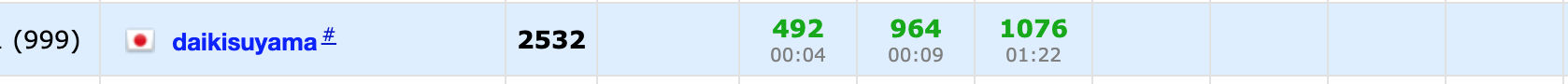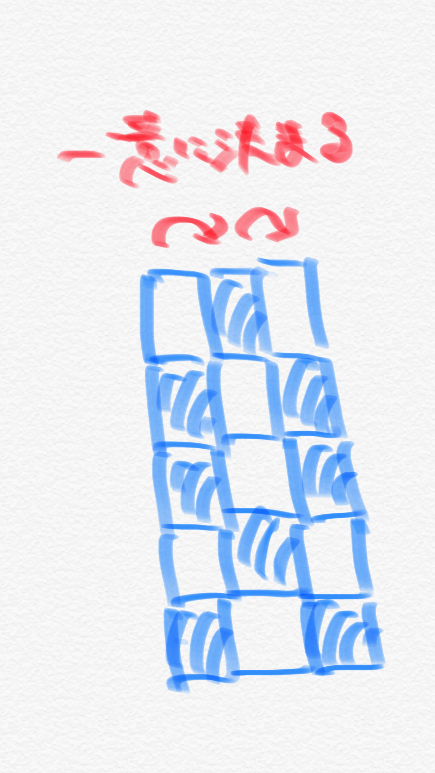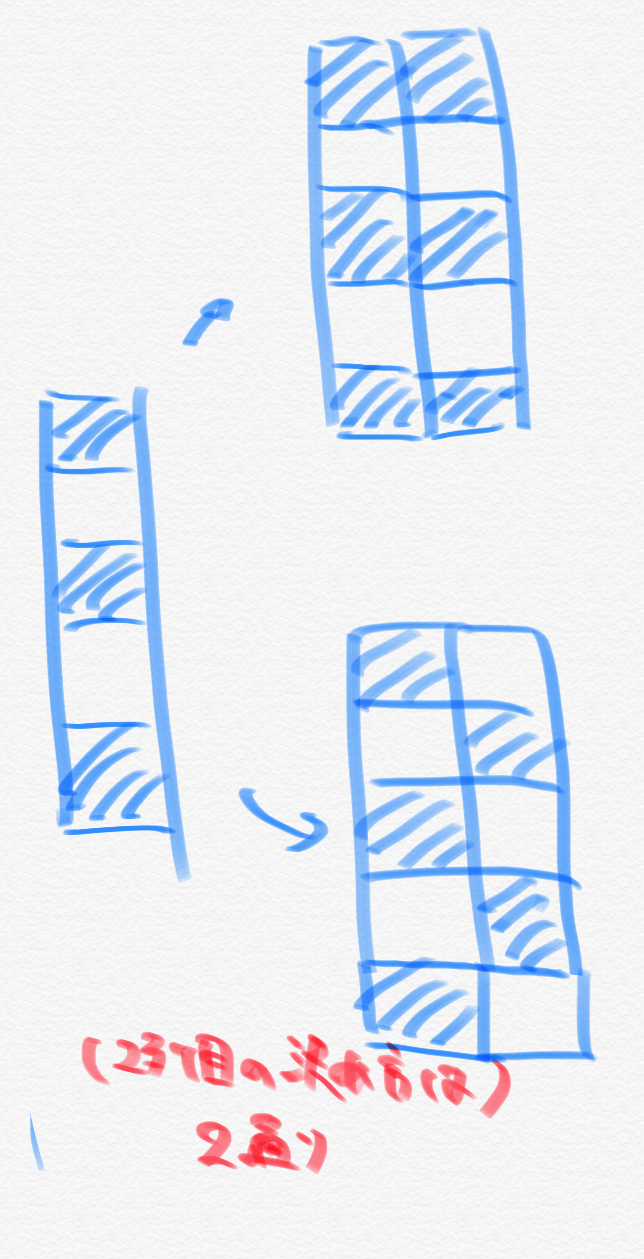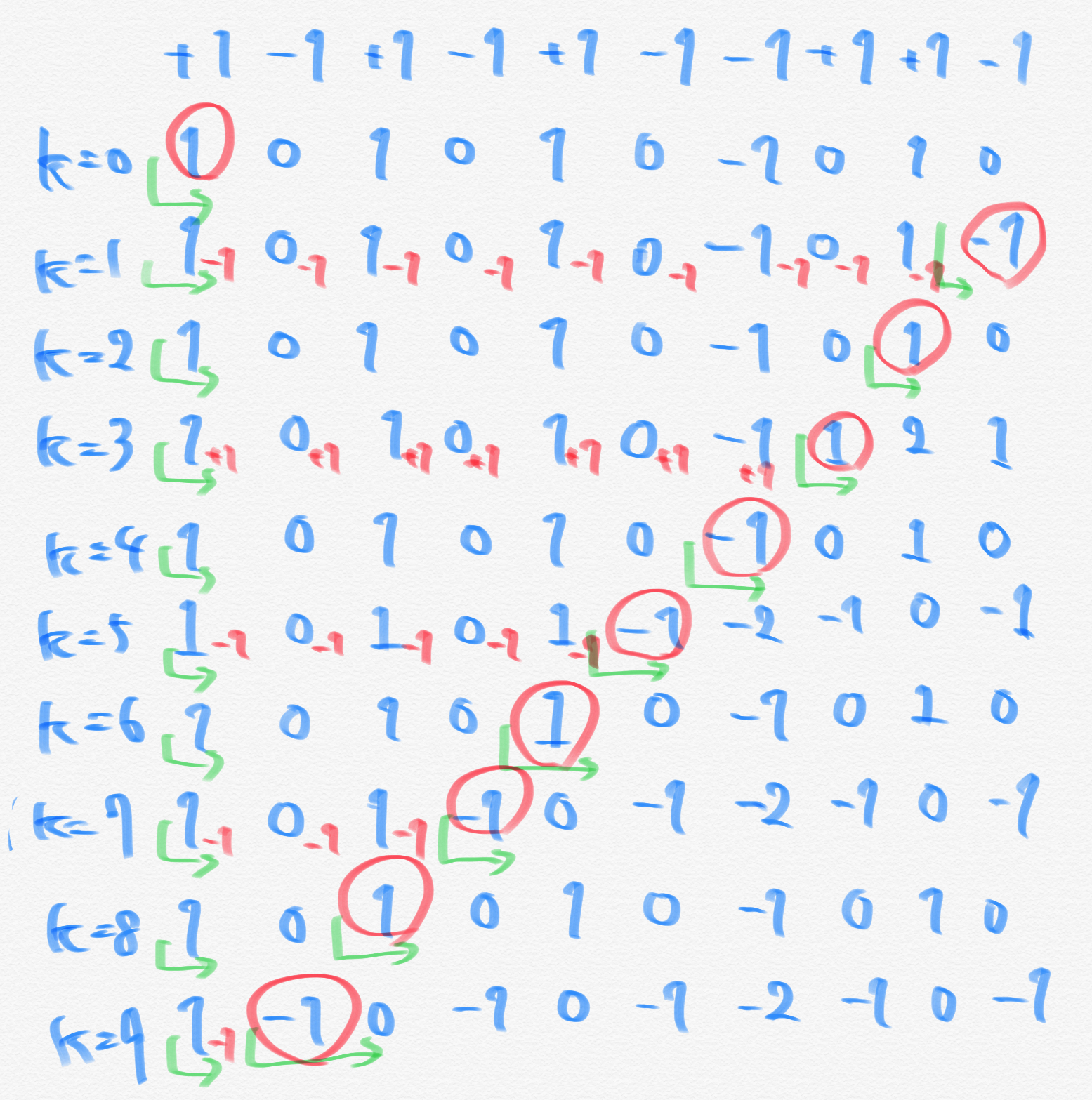# This time's results# Impressions of this time

In D ** I was crying because the subscripts didn't match forever ... As a result, if I solved it after rearranging the policy, I could solve it, so I would like to be aware that ** I will rebuild from the policy if I get stuck in the implementation **.

# Problem A

When you have an intersection, the \$ x \$ coordinates of the intersection will be \$ x + p \ _i = -x + q \ _i \ leftrightarrow x = \ frac {q \ _i-p \ _i} {2} \$. The condition for this to be an integer is when \$ p \ _i, q \ _i \$ is divided by 2 and the remainder is equal. Therefore, (even number contained in \$ p \$) \$ \ times \$ (even number contained in \$ q \$) + (odd number contained in \$ p \$) \$ \ times \$ (included in \$ q \$) The odd number) is the answer.

#### `A.py`

``````
for _ in range(int(input())):
n=int(input())
p=list(map(int,input().split()))
m=int(input())
q=list(map(int,input().split()))
co0=0
for i in range(n):
co0+=(p[i]%2==0)
co1=n-co0
co2=0
for i in range(m):
co2+=(q[i]%2==0)
co3=m-co2
print(co0*co2+co1*co3)
``````

# Problem B

When the final coordinates are \$ (x, y) \$, to maximize \$ x ^ 2 + y ^ 2 \$, you only need to make one of ** \$ x, y \$ as large as possible ** (Here we assume that \$ x \$ is increased). Also, since the sides must be placed at right angles to the side just before, ** up to \$ n- [\ frac {n} {2}] \$ sticks that increase the \$ x \$ coordinates. You can choose as **. Therefore, when sorting the given sticks, the smallest stick to the \$ [\ frac {n} {2}] \$ th smallest stick is selected as the one that increases the \$ y \$ coordinates, and \$ [\ frac {n } {2}] + 1 It is best to choose the stick after the 3rd smallest stick as the one that increases the \$ x \$ coordinates.

#### `B.py`

``````
n=int(input())
a=list(map(int,input().split()))
a.sort()
c,d=0,0
for i in range(n//2):
c+=a[i]
for i in range(n//2,n):
d+=a[i]
print(c**2+d**2)
``````

# C problem

It's a difficult problem, but you can see it by experimenting.

I would like to use DP to handle the condition of 2 consecutive squares, but ** normal 2D DP seems difficult ** because \$ n and m \$ are large. Therefore, I conducted an experiment with the expectation that it would be decided ** to some extent regularly **.

At this time, when I conducted an experiment using samples, I found that ** if the first column was decided, the subsequent columns would be decided in order **. For example, if it is 5 rows and 3 columns, it will be as follows.From the above figure, you can see that by deciding the first column, the columns after that are ** uniquely determined **. You can also see that the ** odd-numbered and even-numbered columns have the same pattern **.

However, there are some patterns ** that cannot be uniquely determined. That is, it is a pattern in which black and white cells are staggered as shown below. There may be more than one of the following columns for this pattern: (I will not prove here that there are not multiple ways in other patterns, but it can be proved by using the fact that it is uniquely determined in the case of cells with consecutive colors)Also, when the pattern in which the black squares are in the first row is A and the pattern in which the white squares are in the first row is B, in the case of ** AA or BB, the next columns are B and A, respectively. Must be **. Based on the above, after finding the number of black and white squares in the first row, the number of rows in the pattern of alternating black and white is calculated separately. You can see that you can write a program of O (n + m) \$.

I spent too much time because I couldn't move on to the implementation after summarizing the considerations so far. It is a reflection. Anyway, we will consider the implementation below. You also need to remember to divide by \$ 10 ^ 9 + 7 \$.

① How to decide the first row

When black and white alternate in the first row, there are always two ways, which are counted in ② (hence, ** excluded here **). For other patterns, the columns after that are uniquely determined. Therefore, consider the following DP to see how many black and white cells are arranged in the first row (white when \$ j = 0 \$, black when \$ j = 1 \$, \$ k = 0,1 \$). is).

\$ dp [i] [j] [k]: = \$ (Number of cells arranged when \$ k + 1 \$ cells continue when the \$ i \$ line is determined)

At this time, the transition is as follows (abbreviated).

(1) When \$ k = 0 \$ You can choose either black or white squares, so you can think of two transitions from \$ dp [i] [j] [k] \$.

(2) When \$ k = 1 \$ There can only be one way to choose a different color square from \$ dp [i] [j] [k] \$, as it must not be more than two in a row.

After considering the transition, (the sum of \$ dp [n-1] \$) -2 is the answer.

(2) There are several ways to decide when the pattern is a pattern in which black and white squares alternate in the first row.

As before, if you consider the pattern in which the black squares are in the top row as A and the pattern in which the white squares are in the top row as B, you can set the following DP (\$ j = 0). When \$, A, \$ j = 1 \$, B, \$ k = 0,1 \$).

\$ dp [i] [j] [k]: = \$ (Number of columns arranged when \$ k + 1 \$ patterns of \$ j \$ continue when the \$ i \$ column is determined)

At this time, the transition is as follows (it is abbreviated a little).

(1) When \$ k = 0 \$ You can choose either pattern A or B, so you can think of two transitions from \$ dp [i] [j] [k] \$.

(2) When \$ k = 1 \$ There can only be one way to choose a different pattern column from \$ dp [i] [j] [k] \$, as it must not be more than two consecutive.

After considering the transition, (the sum of \$ dp [n-1] \$) is the answer.

As mentioned above, the total number of patterns of ① and ② should be calculated as the answer. Also, you can see that the DP performed in ① and ② is the same.

#### `C.py`

``````
mod=10**9+7
n,m=map(int,input().split())
n,m=min(n,m),max(n,m)
dp=[[[0,0] for j in range(2)] for i in range(m)]
dp=[[1,0],[1,0]]
for i in range(m-1):
for j in range(2):
for k in range(2):
if j==0 and k==0:
dp[i+1][j]+=dp[i][j][k]
dp[i+1][j+1]+=dp[i][j][k]
elif j==1 and k==0:
dp[i+1][j]+=dp[i][j][k]
dp[i+1][j-1]+=dp[i][j][k]
elif j==0 and k==1:
dp[i+1][j+1]+=dp[i][j][k]
else:
dp[i+1][j-1]+=dp[i][j][k]
for j in range(2):
for k in range(2):
dp[i+1][j][k]%=mod
ans=-2
for j in range(2):
for k in range(2):
ans+=dp[m-1][j][k]
ans%=mod
dp2=[[[0,0] for j in range(2)] for i in range(n)]
dp2=[[1,0],[1,0]]
for i in range(n-1):
for j in range(2):
for k in range(2):
if j==0 and k==0:
dp2[i+1][j]+=dp2[i][j][k]
dp2[i+1][j+1]+=dp2[i][j][k]
elif j==1 and k==0:
dp2[i+1][j]+=dp2[i][j][k]
dp2[i+1][j-1]+=dp2[i][j][k]
elif j==0 and k==1:
dp2[i+1][j+1]+=dp2[i][j][k]
else:
dp2[i+1][j-1]+=dp2[i][j][k]
for j in range(2):
for k in range(2):
dp2[i+1][j][k]%=mod
for j in range(2):
for k in range(2):
ans+=dp[n-1][j][k]
ans%=mod
print(ans)
``````

# D1 problem

This is a problem that could not be considered and organized. It's a shame because it's the kind of problem I don't want to drop the most.

In this problem, \$ N \$ is up to about 500, so you can see that \$ O (N ^ 3) \$ is okay and \$ O (N ^ 4) \$ is not. Now, let's say you swap between the \$ i \$ th and the \$ j \$ th, and you can try all ** cyclical shifts with \$ O (N) \$ for that parenthesis string **.

Here, the condition for establishing the parenthesized string is that ** "(" is +1, ")" is regarded as -1 and the cumulative sum is always 0 or more and the last element is 0 **. Even if you swap here, the number of "(" and ")" does not change, so you only need to check ** whether the value of the element that minimizes the cumulative sum is 0 or more **. Also, the cyclical shift is easy to understand by looking at it while shifting the first element as shown below (pattern in sample 1).Therefore, ** it is good if it is always 0 or more on the right side or the left side when performing a cyclical shift **. This means that if the cumulative sum taken from the front is sum1, the cumulative sum taken from the back is sum2, the cumulative minimum taken from the front is amin1, and the cumulative minimum taken from the back is amin2, the index at the leftmost position is \$. When k \$, the condition on the right side is \$ amin2 [k + 1] -asum1 [k] \ geqq 0 \$ and the condition on the left side is \$ amin1 [k + 1] + asum2 [k] \ geqq 0 \$. When any of these conditions are met, it can be said that the parenthesized sequence is a cyclical shift (✳︎).

From the above, it is a sufficiently fast program because it is \$ O (N) \$ for the pre-calculation of the cumulative sum and the judgment in each cyclical shift.

(✳︎)… My head broke in the cumulative part, but it's not that difficult….

#### `D.cc`

``````

//Compiler optimization
#pragma GCC optimize("Ofast")

//Include etc.
#include<bits/stdc++.h>
using namespace std;
typedef long long ll;

//macro
//for loop
//The argument is(Variables in the loop,Range of movement)Or(Variables in the loop,First number,Number of endings)、のどちらOr
//If there is no D, the loop variable is incremented by 1, and if it is with D, the loop variable is decremented by 1.
//FORA is a range for statement(If it's hard to use, erase it)
#define REP(i,n) for(ll i=0;i<ll(n);i++)
#define REPD(i,n) for(ll i=n-1;i>=0;i--)
#define FOR(i,a,b) for(ll i=a;i<=ll(b);i++)
#define FORD(i,a,b) for(ll i=a;i>=ll(b);i--)
#define FORA(i,I) for(const auto& i:I)
//x is a container such as vector
#define ALL(x) x.begin(),x.end()
#define SIZE(x) ll(x.size())
//constant
#define INF 1000000000000 //10^12:∞
#define MOD 1000000007 //10^9+7:Congruence law
#define MAXR 100000 //10^5:The largest range in the array
//Abbreviation
#define PB push_back //Insert
#define MP make_pair //pair constructor
#define F first //The first element of pair
#define S second //The second element of pair

signed main(){
//Output specification of decimal digits
//cout<<fixed<<setprecision(10);
//Code for speeding up input
//ios::sync_with_stdio(false);
//cin.tie(nullptr);
ll n;cin>>n;
string s;cin>>s;
ll ma=0;pair<ll,ll> now={1,1};
if(n%2!=0 or ll(count(ALL(s),'('))!=ll(n/2)){
cout<<0<<endl;
cout<<"1 1"<<endl;
return 0;
}
vector<ll> checks(n);
REP(i,n){
if(s[i]=='('){
checks[i]=1;
}else{
checks[i]=-1;
}
}
REP(i,n){
REP(j,n){
vector<ll> check=checks;
swap(check[i],check[j]);
//Take the cumulative sum from the front
vector<ll> asum1(n);asum1=check;
REP(k,n-1)asum1[k+1]=asum1[k]+check[k+1];
//Take the cumulative sum from behind
vector<ll> asum2(n);asum2[n-1]=check[n-1];
REPD(k,n-1)asum2[k]=asum2[k+1]+check[k];
//Take the cumulative minimum from the front(Cumulative sum)
vector<ll> amin1(n);amin1=asum1;
REP(k,n-1)amin1[k+1]=min(amin1[k],asum1[k+1]);
//Take the cumulative minimum from behind(Cumulative sum)
vector<ll> amin2(n);amin2[n-1]=asum1[n-1];
REPD(k,n-1)amin2[k]=min(amin2[k+1],asum1[k]);

ll ans=0;
if(amin1[n-1]>=0)ans++;
REP(k,n-1){
if(amin2[k+1]-asum1[k]>=0 and amin1[k]+asum2[k+1]>=0){
ans++;
}
}
if(ma<ans){
ma=ans;
now={i+1,j+1};
}
}
}
cout<<ma<<endl;
cout<<now.F<<" "<<now.S<<endl;
}
``````

# After the D2 problem

I will not solve this time.